“DeepLab: Deep Labelling for Semantic Image Segmentation” is a state-of-the-art deep learning model from Google for sementic image segmentation task, where the goal is to assign semantic labels (e.g. person, dog, cat and so on) to every pixel in the input image.

# Summary

• Motivation
• In order to capture the contextual information at multiple scales, DeepLabv3 applies several parallel atrous convolution with different rates (called Atrous Spatial Pyramid Pooling, ASPP)
• Problem: Even though rich semantic information is encoded in the last feature map, detailed information related to object boundaries is missing due to the pooling or convolutions with striding operations within the network backbone.
• Solution: This could be alleviated by applying the atrous convolution to extract denser feature maps.
• Problem: However, it is computationally prohibitive to extract output feature maps.
• Solution: On the other hand, encoder-decoder models lend themselves to faster computation in the encoder path and gradually recover sharp object boundaries in the decoder path.
• This paper attempts to combine the advantages from both methods.
• Proposed Solution
• Extends DeepLabv3 by adding a simple yet effective decoder module to recover the object boundaries.
• The rich semantic information is encoded in the output of DeepLabv3, with atrous convolution allowing one to control the density of the encoder features.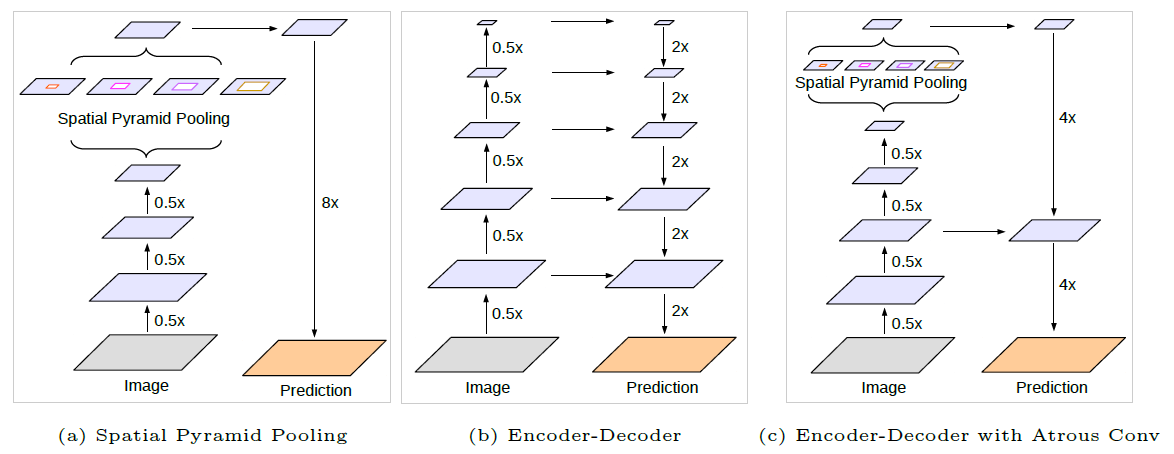Figure 1: This paper improves DeepLabv3, which employs the spatial pyramid pooling module (a), with the encoder-decoder structure (b). The proposed model, DeepLabv3+, contains rich semantic information from the encoder module, while the detailed object boundaries are recovered by the simple yet effective decoder module. The encoder module allows us to extract features at an arbitrary resolution by applying atrous convolution.

• Contributions
• Proposed a novel encoder-decoder structure which employs DeepLabv3 as a powerful encoder module and a simple yet effective decoder module.
• In the proposed structure, one can arbitrarily control the resolution of extracted encoder features by atrous convolution to trade-off precision and runtime.
• Adapt the Xception model for the segmentation task and apply depthwise separable convolution to both ASPP module and decoder module, resulting in a faster and stronger encoder-decoder network.
• Proposed model attains a new state-of-art performance on PASCAL VOC 2012 and Cityscapeds datasets.

# Related Work

## Spatial Pyramid Pooling

• PSPNet or DeepLab perform spatial pyramid pooling at several grid scales or apply several parallel atrous convolution with different rates.
• Captures rich contextual information and multi-scale information by pooling features at different resolution.

## Encoder-Decoder

• Typically, the encoder-decoder networks contain
• An encoder module that gradually reduces the feature maps and captures higher semantic information
• A decoder module that gradually recovers the spatial information
• This paper propose to use DeepLabv3 as the encoder module and add a simple yet effective decoder module to obtain sharper segmentations.

## Depthwise Separable Convolution

• Depthwise separable convolution or group convolution, a powerful operation to reduce the computation cost and number of parameters while maintaining similar performance.

# Methods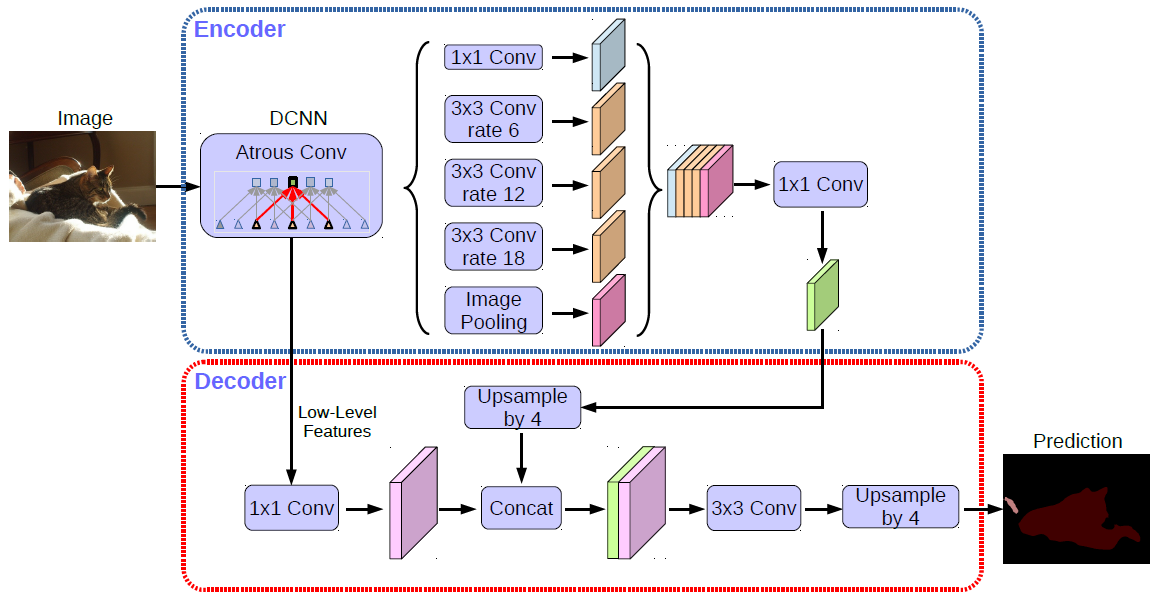Figure 2: DeepLabv3+ extends DeepLabv3 by employing a encoder-decoder structure. The encoder module encodes multi-scale contextual information by applying atrous convolution at multiple scales, while the simple yet effective decoder module refines the segmentation results along object boundaries.

## Atrous Convolution

• It allows us to explicitly control the resolution of features computed by deep CNNs
• And adjust filter’s field-of-view in order to capture multi-scale information.
• Atrous rate r determines the stride with which we sample the input signal.
• The standard convolution is a special case in which rate r=1.

## Depthwise Separable Convolution

• Depthwise Convolution
1. Split the input into channels, and split the filter into channels (the number of channels between input and filter must match).
2. For each of the channels, convolve the input with the corresponding filter, producing an output tensor (2D).
3. Stack the output tensors back together.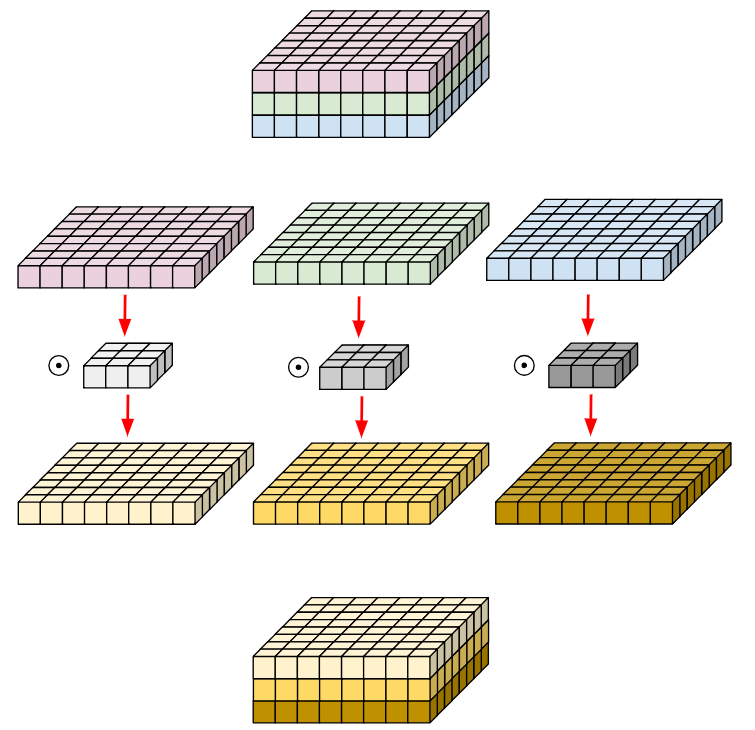Figure 3: Depthwise convolution.

• Depthwise separable convolution, factorizing a standard convolution into a depthwise convolution followed by a pointwise convolution (1x1 conv), drastically reduces computation complexity.
• Specifically, the depthwise convolution performs a spatial convolution independently for each input channel
• While the pointwise convolution is employed to combine the output from the depthwise convolution.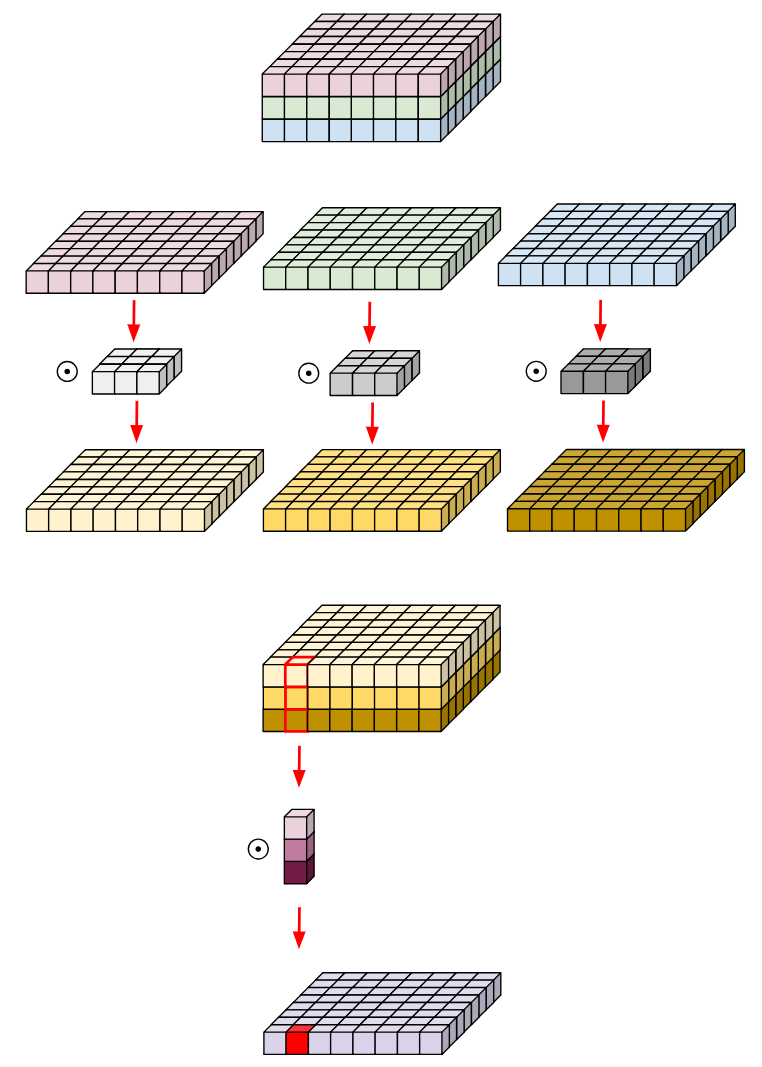Figure 4: Depthwise seperable convolution.

• Atrous separable convolution significantly reduces the computation complexity of proposed model while maintaining similar (or better) performance.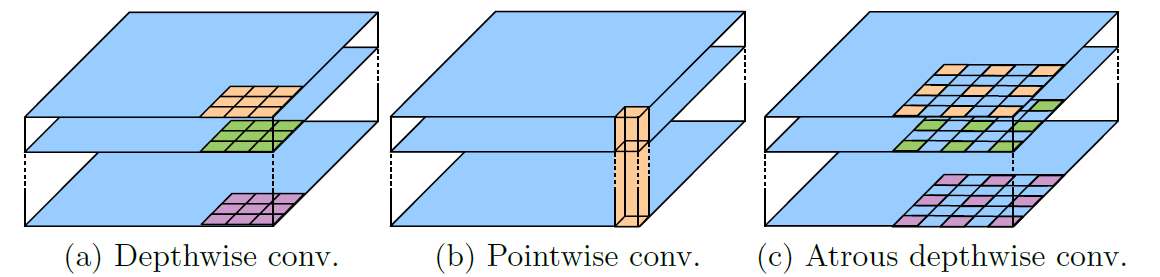Figure 5: 3X3 Depthwise separable convolution decomposes a standard convolution into (a) a depthwise convolution (applying a single filter for each input channel) and (b) a pointwise convolution (combining the outputs from depthwise convolution across channels). In this work, we explore atrous separable convolution where atrous convolution is adopted in the depthwise convolution, as shown in (c) with rate = 2.

## DeepLabv3 as Encoder

• DeepLabv3+ uses DeepLabv3 as encoder which employs atrous convolution to extract the features computed by deep CNN at an arbitrary resolution
• Output stride: the ratio of input image spatial resolution to the final output resolution.
• For the task of semantic segmentation, one can adopt output stride = 16 or 8 for denser feature extraction by removing the striding in the last blocks and applying the astrous convolution correspondingly.

## Proposed Decoder

• The encoder features are first bilinearly upsampled by a factor of 4 and then concatenated with the corresponding low-level features from the network backbone that have the same spatial resolution.
• Apply another 1x1 convolution on the low-level features to reduce the number of channels, since the corresponding low-level features usually contain a large number of channels.
• In the experiments, using output stride = 16 for the encoder module strikes the best trade-off between speed and accuracy.
• The performance is marginally improved when using output stride = 8 for the encoder module at the cost of extra computation complexity.

## Modified Aligned Xception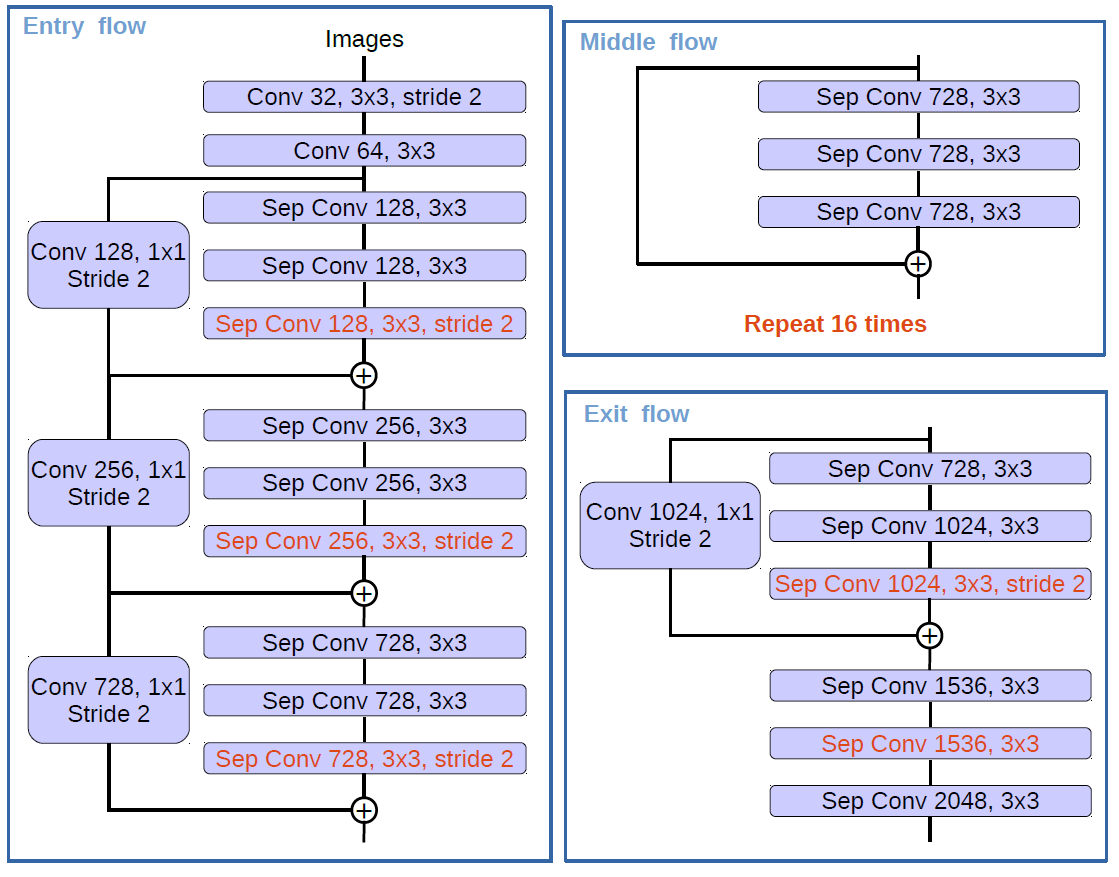Figure 6: The authors modify the Xception as follows: (1) more layers (same as MSRA’s modification except the changes in Entry ow), (2) all the max pooling operations are replaced by depthwise separable convolutions with striding, and (3) extra batch normalization and ReLU are added after each 3 x 3 depthwise convolution, similar to MobileNet.

## Experimental Evaluation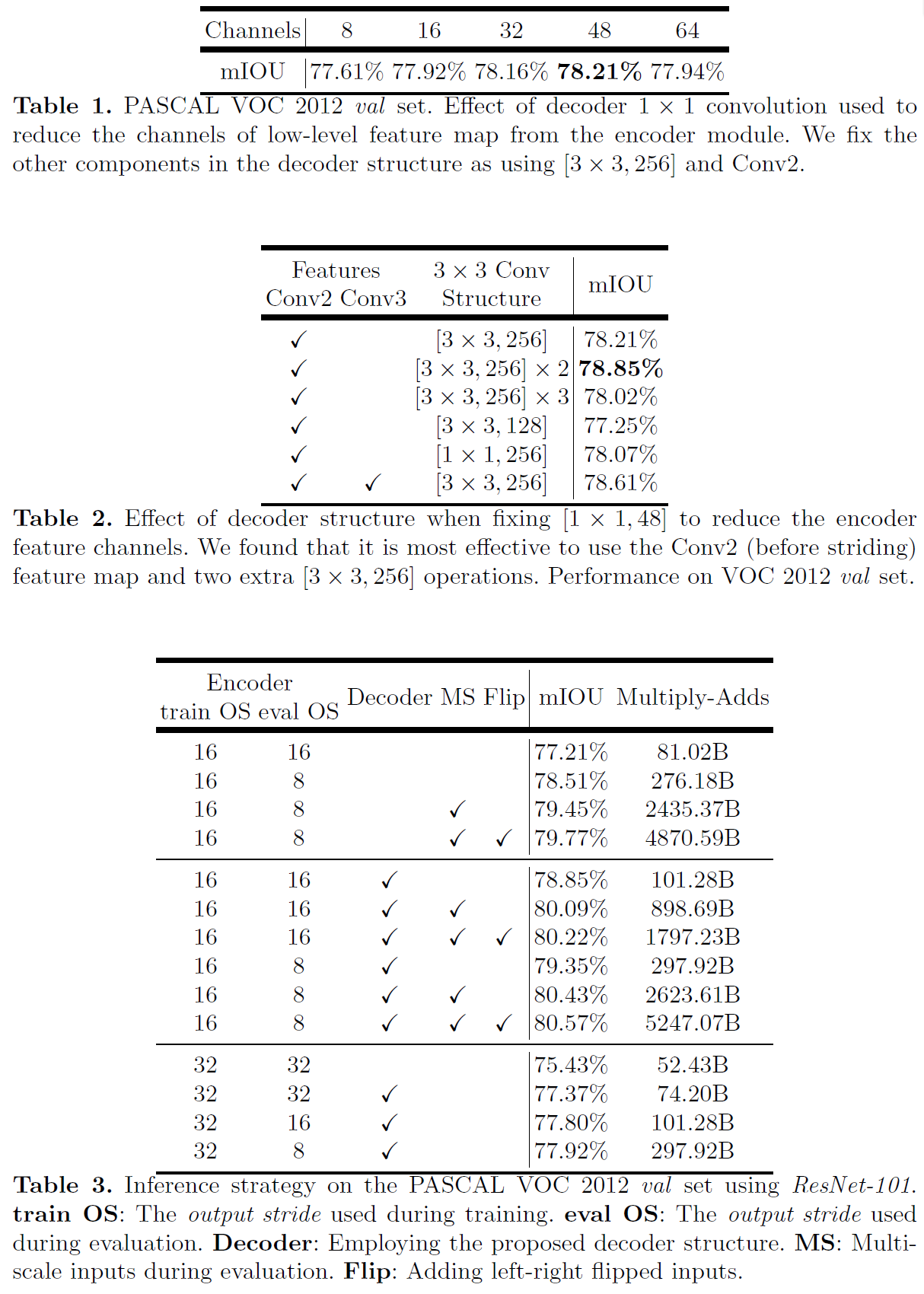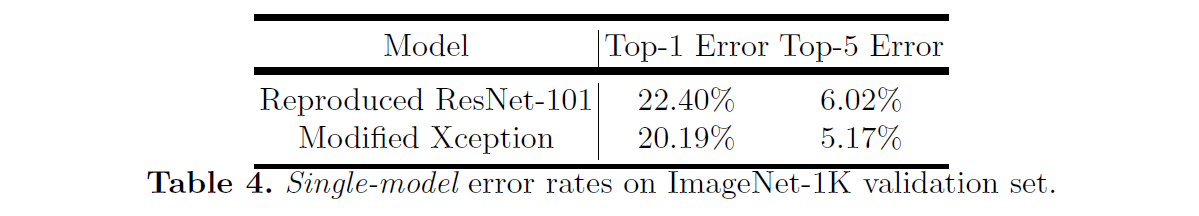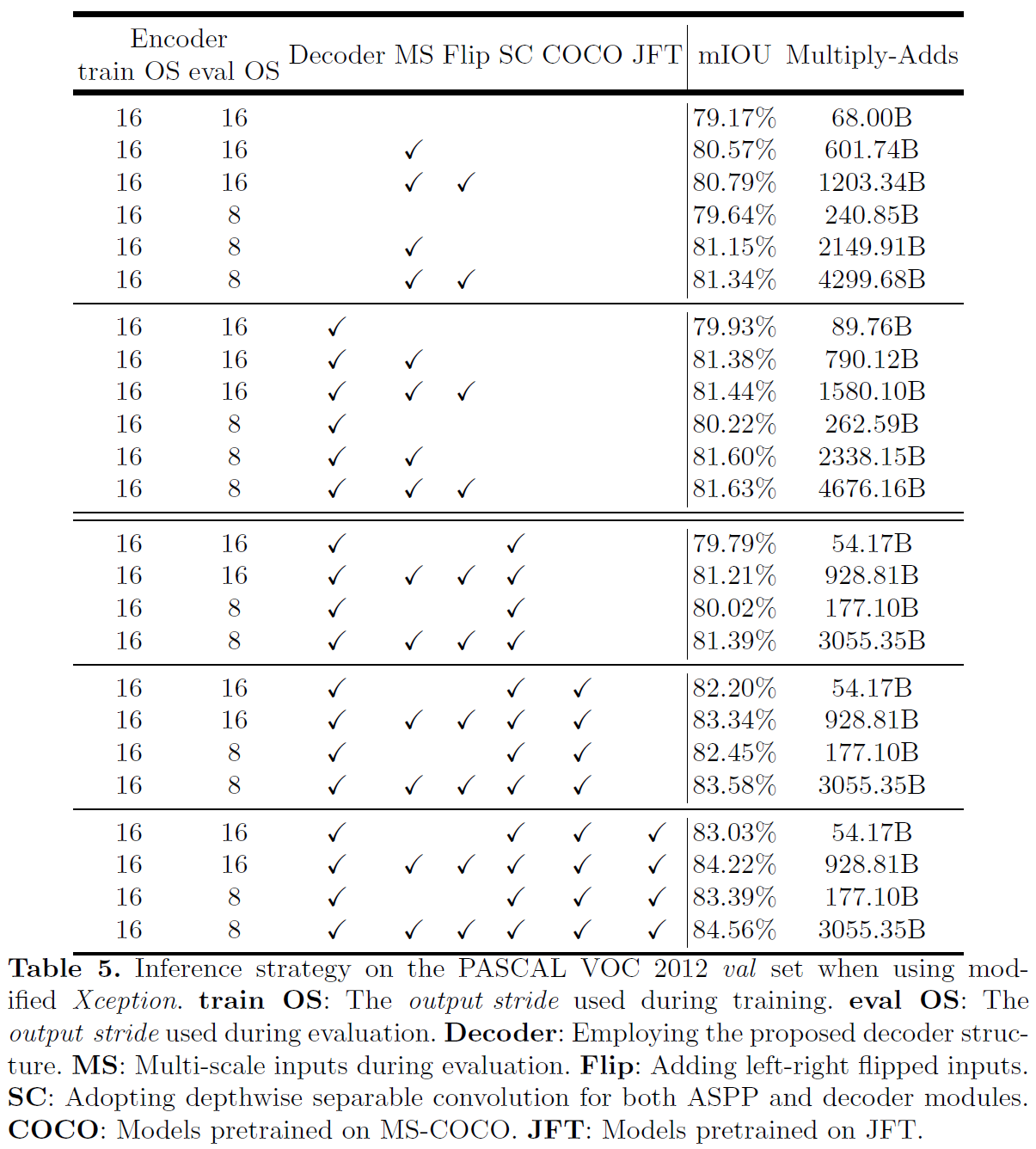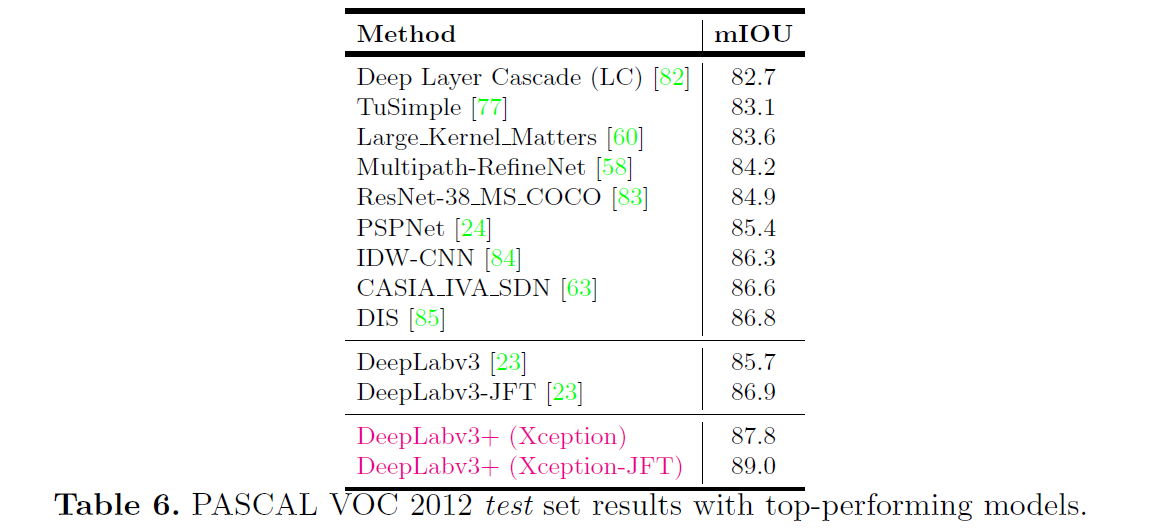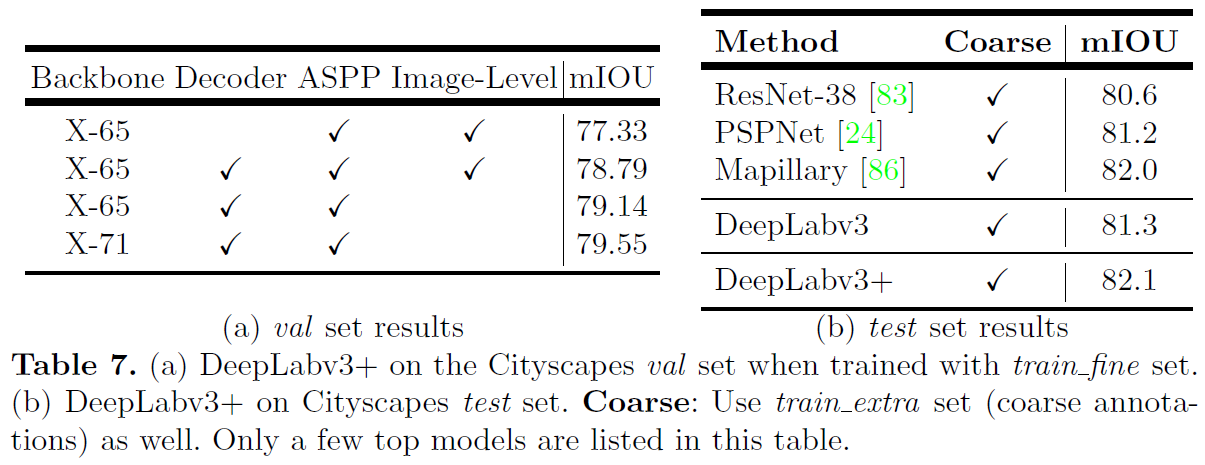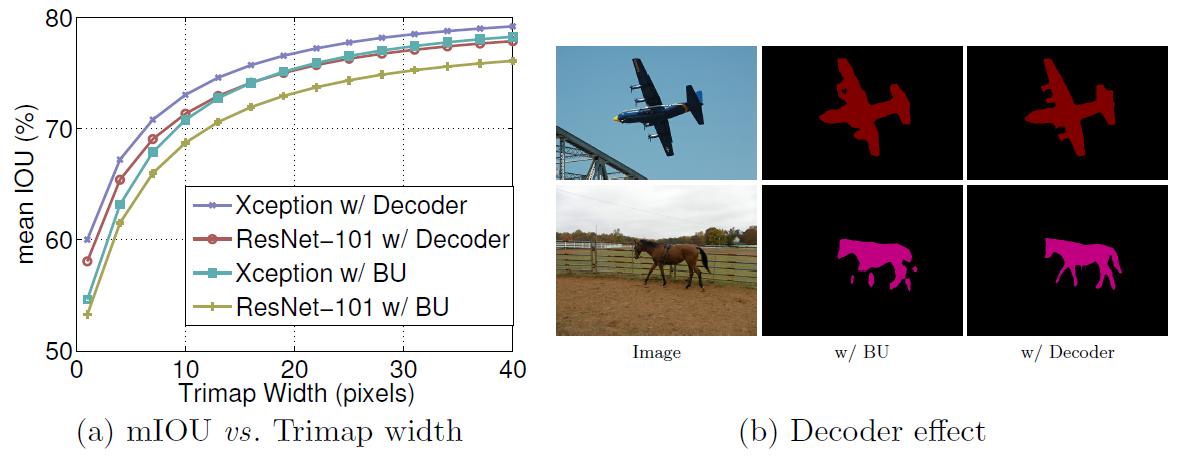Figure 6: (a) mIOU as a function of trimap band width around the object boundaries when employing train output stride = eval output stride = 16. BU: Bilinear upsampling. (b) Qualitative effect of employing the proposed decoder module compared with the naive bilinear upsampling (denoted as BU). In the examples, we adopt Xception as feature extractor and train output stride = eval output stride = 16.